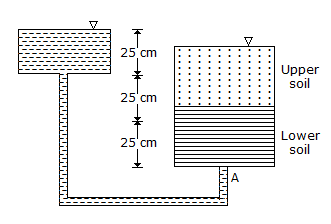# Civil Engineering - UPSC Civil Service Exam Questions

21.

Consider the following statements :
I: To insert a 28 mm nominal diameter rivet, 29.5 mm rivet hole is made.
II: Provision is made to allow temperature expansion of the bolt.

 A. both I and II are true B. I is true but II is false C. I is false but II is true D. both I and II are false

Explanation:

No answer description available for this question. Let us discuss.

22.

Consider the following statements :
A : For beams, I-sections are more economical than rectangular section.
R : Most of the material is concentrated away from the centroid.
Of these statements :

 A. Both A and R are true and R is the correct explanation of A B. Both A and R are true but R is not a correct explanation of A C. A is try but R is false D. A is false but R is true.

Explanation:

No answer description available for this question. Let us discuss.

23.

Two different granular soils are placed in a permeameter tube and flow is allowed to take place under a constant total head. The total head and pressure head at point A in centimeters, are respectively.A. 75, 75 B. 25, 75 C. 25, 25 D. 75, 25

Explanation:

No answer description available for this question. Let us discuss.

24.

When the degree of consolidation is 50%, the time factor is about

 A. 0.2 B. 0.5 C. 1 D. 2

Explanation:

No answer description available for this question. Let us discuss.

25.

General shrinkage in cement concrete is caused by

 A. carbonation B. stresses due to external load C. drying the starting with a stiff consistency D. drying starting with a wetter consistency.